# FIRST LAW OF THERMODYNAMICS: CONSERVATION OF ENERGY

## First Law of Thermodynamics (VW, S & B: 2.6)

1. ### There exists for every system a property called energy. E = internal energy (arising from molecular motion - primarily a function of temperature) + kinetic energy + potential energy + chemical energy.

1. Defines a useful property called "energy".

2. The two new terms in the equation (compared to what you have seen in physics and dynamics, for example) are the internal energy and the chemical energy. For most situtations in this class, we will neglect the chemical energy.

3. Let’s focus on the internal energy, u. It is associated with the random or disorganized motion of the particles.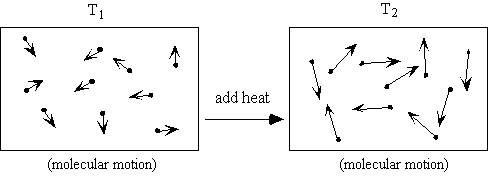u is a function of the state of the system. Thus u = u (p, T), or u = u (p, v), or u = u(v,T). Recall that for pure substances the entire state of the system is specified if any two properties are specified. We will discuss the equations that relate the internal energy to these other variables later in the class.

## DE = Q - W (units are Joules)

1. The signs are important (and sometimes confusing!)

2. E is the energy of the system

Q is the heat transferred to the system (positive) - if it is transferred

from the system Q is negative. (VW, S & B: 4.7-4.8)

W is the work done by the system (positive) - if work is done on

the system W is negative. (VW, S & B: 4.1-4.4)

3. The equation can also be written on a per unit mass basis

4. De = q - w (units are J/kg)

5. In many situations the potential energy and the kinetic energy of the system are constant.
6. Then De = Du, and

Du = q - w or DU = Q - W

Q and W are path dependent, U is not it depends only on the state of the system, not how the system got to that state.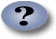7. Can also write the first law in differential form:

8. dU = dQ - dW or du = dq - dw

Here the symbol "d" is used to denote that these are not exact differentials but are dependent on path.

9. Or for quasi-static processes

10. dU = dQ - pdV or du = dq - pdv

11. Example: Heat a gas, it expands against a weight. Force (pressure times area) is applied over a distance, work is done.

12.13. We will see later that the First Law can be written for a control volume with steady mass flow in and steady mass flow out (like a jet engine for example). We will call this the Steady-Flow Energy Equation. (VW, S & B: 5.8-5.12)

14. We will spend most of the course dealing with various applications of the first law - in one form or another.

## Corollaries of the First Law

### Work done in any adiabatic (Q=0) process is path independent.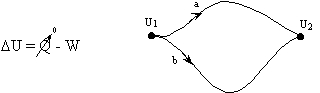### For a cyclic process heat and work transfers are numerically equal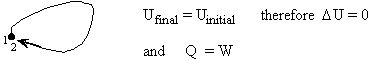or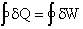## Example applications of the First Law to motivate the use of a property called "enthalpy" (VW, S & B: 5.4-5.5)

### The combination u+pv shows up frequently so we give it a name: "enthalpy" h= u+pv (or H = U+pV). It is a function of the state of the system.

The utility and physical significance of enthalpy will be clearer when we discuss the steady flow energy equation in a few lectures. For now, you may wish to think of it as follows (Levenspiel, 1996). When you evaluate the energy of an object of volume V, you have to remember that the object had to push the surroundings out of the way to make room for itself. With pressure p on the object, the work required to make a place for itself is pV. This is so with any object or system, and this work may not be negligible. (Recall, the force of one atmosphere pressure on one square meter is equivalent to the force of a mass of about 10 tons.) Thus the total energy of a body is its internal energy plus the extra energy it is credited with by having a volume V at pressure p. We call this total energy the enthalpy, H.

### Consider a quasi-static process of constant pressure expansion

Q = (U2 - U1) +W

= (U2 - U1) + p(V2 - V1) since p1 = p2 = p

so Q = (U2 + pV2) - (U1 + pV1) = H2 - H1

### Consider adiabatic throttling of a gas (gas passes through a flow resistance). What is the relation between conditions before and after the resistance?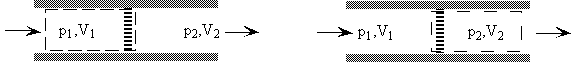Q = 0 therefore DU = - DW

or U2 - U1 = -(p2V2 - p1V1)

so U2 + p2V2 = U1 + p1V1

H2 = H1

## First Law in terms of enthalpy

dU = dQ - dW (for any process, neglecting DKE and DPE)

dU = dQ - pdV (for any quasi-static process, no DKE or DPE)

H = U + pV therefore dH = dU + pdV + Vdp

so dH = dQ - dW + pdV + Vdp (any process)

or

dH = dQ + Vdp (for any quasi-static process)

## Specific Heats and Heat Capacity (VW, S & B: 5.6)

### Q = CDT

where C is a constant that depends on the substance.

1. For a constant pressure process:

2.or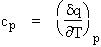and for a constant volume process: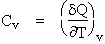or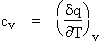we use cp and cv to relate u and h to the temperature for an ideal gas.

3. Expressions for u and h. Remember that if we specify any two properties of the system, then the state of the system is fully specified. In other words we can write u = u(T,v), u=u(p,v) or u=u(p,T) -- the same holds true for h. (VW, S & B: 5.7)

Consider a constant volume process and write u = u(T,v). Thenwhere the last term is zero since there is no change in volume. Now if we write the First Law for a quasi-static process

du = dq - pdv

where again the last term is zero since there is no volume change. So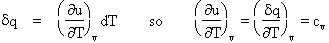so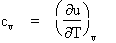If we write h=h(T,p), and consider a constant pressure process, we can perform similar manipulations and show that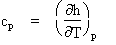cp and cv are thermodynamic properties of a substance. The previous two relationships are valid at any point in any quasi-static process whether that process is constant volume, constant pressure, or neither.### Ideal gas assumption (VW, S & B: 5.7)

If we have a thermally perfect gas (i.e. it obeys pv=RT), then it is called an ideal gas if u = u(T) only, and h= h(T) only. Then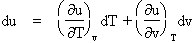andso for an ideal gas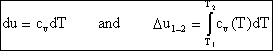andOver small temperature changes (DT Å 200K), it is often assumed that cp and cv are constant.

For an ideal gas, du=cvdT and dh=cpdT for any process (whether constant volume or constant pressure or not). This is an empirical fact for these special substances. If this is difficult to understand, put yourself in the position of an experimentalist who is attempting to measure cv for a substance. For such an experiment, you might add a known amount of heat to a rigid volume and measure the temperature change. Imagine repeating the experiment several times and getting the exact same answer, but one day finding out that during some of the repeats the volume was changing. This would be unusual and special and you might give this substance a special name. The special name we use for these substances is "ideal gas". (The same is true for cp. You might use a low friction piston-cylinder arragement with a fixed weight to maintain constant pressure while adding heat and measuring the temperature. Then one day you find that piston was getting stuck so the pressure was not constant afterall, but you still obtained the same temperature rise for the same amount of heat addition.)

### First Law Expressions for an Ideal Gas

1. For an ideal gas undergoing a quasi-static process:

2. dq = cvdT + pdv

or in terms of enthalpy

dq = cpdT - vdp

3. Relationships between thermodynamic properties cv, cp, and R

1. Equating the two first law expressions given above

2. cpdT - vdp = cvdT + pdv

(cp - cv)dT = d(pv)

cp - cv = d(pv)/dT and pv = RT

so

cp - cv = R

3. The ratio of specific heats, g

g = cp/cv

4. Example: Quasi-static, adiabatic process for an ideal gas

dq = cvdT + pdv and dq = cpdT - vdp

so

cv dT = -pdv and cp dT = vdp

therefore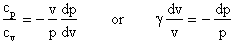then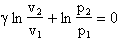or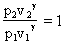finally, we arrive at the very useful expression

pvg = constant

from which it can also be shown that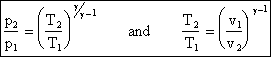We will use the above equation to relate pressure and temperature to one another for quasi-static adiabatic processes (our idealization of what happens in compressors and turbines).### Questions:

1. On a p-v diagram for a closed-system sketch the thermodynamic paths that the system would follow if expanding from volume = v1 to volume = v2 by isothermal and quasi-static, adiabatic processes.

2. For which process is the most work done by the system?

3. For which process is there heat exchange? Is it added or removed?

4. Is the final state of the system the same after each process?

5. Derive expressions for the work done by the system for each process.